# The SPP Procedure

#### EDF Goodness-of-Fit Tests

You use goodness-of-fit tests to examine the fit of a parametric distribution. In the SPP procedure, this task emerges when you test your data for dependence on a covariate. You can examine the goodness of fit by using tests that are based on the EDF. These tests offer advantages over traditional chi-square goodness-of-fit tests, as discussed in D’Agostino and Stephens (1986). The empirical distribution function is defined for a set of n independent observations,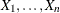, that have a common distribution function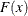as follows. Denote the observations ordered from smallest to largest as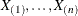. Then the empirical distribution function,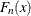, is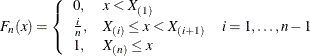is a step function that takes a step of heightat each observation. This function estimates the distribution function. At any value x,is the proportion of observations that are less than or equal to x, whereasis the probability of an observation being less than or equal to x. EDF statistics measure the discrepancy betweenand.

The computational formulas for the EDF statistics make use of the probability integral transformation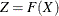. If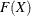is the true distribution function of X, then the random variable Z is uniformly distributed between 0 and 1. For example, assume that you believe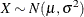. In this case, the probability integral transformfor the normal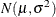is given by the EDF of the standardized value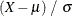. To test the fit of your sample EDFto the assumed exact, you can equivalently test the fit of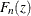to the EDF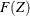of Z. As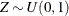,is the cumulative density function (CDF) of the standard uniform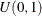, which is simply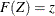. This also means that your empirical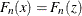. Consequently, the probability integral transform translates the initial fit task into an easier comparison betweenand.

There are two main classes of EDF statistics: the supremum and the quadratic class. The supremum class is based on the largest vertical difference betweenand. The quadratic class is based on the squared difference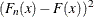. Quadratic statistics have the following general form: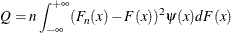The function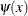weights the squared difference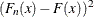.

As previously discussed, the SPP procedure considers the ordered observationsand computes the values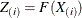by applying the probability integral transform. PROC SPP examines the goodness of fit by computing the following two EDF statistics:

• Kolmogorov-Smirnov two-sided D from the supremum class

• Cramér-von Mises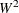from the quadratic class

Within the different classes of EDF statistics, the quadratic class is known to have more powerful statistics than the supremum class. The details of the statistics used by PROC SPP are discussed in the following subsection.

After the EDF test statistics are computed, the SPP procedure computes the associated significance values. In the scope of the PROC SPP analysis, the true distribution function,, is a completely specified distribution. For computations in this scenario, PROC SPP applies slightly modified D andstatistics, as described by D’Agostino and Stephens (1986).